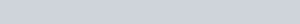1. Nina has to study Math for her Selective Test. She has decided to dedicate 1 hour 30 mins for each topic starting from 7:30 pm. Considering she studies 3 topics a day with a 10 mins break in between each topic, find out by when she will be done by the second topic.
a. 9:10 pm
b. 11:20 pm
c. 10:30 pm
d. 10:40 pm
e. 11:40 pm.

Explanation: It is 10:40 as work from 7:30, add 1 and a half hour for the first topic, then add 10 minutes for the break, and lastly add 1 and a half hours of the second topic, you will get 10:40.

2. Kevin loves playing with numbers. He has to divide the largest 3-digit number with the smallest multiple of 9. What is the reminder he gets?

a. 1
b. 9
c. 0
d. 2
e. 4

Explanation: The answer is ‘0’ as the largest three-digit number is: 999. The smallest multiple of ‘9’ is 9. So 999 divided by 9 is 111, with zero remainder.

3. What is the angle made between the minute and the hour hand when the hour hand is at 5 o’clock?

a. 130˚
b. 150˚
c. 125˚
d. 180˚
e. 165˚

Explanation: 30 degree times 5 =150 degree

4.In the above figure, A and B are two points 1000 m apart. After some time, A moves by 700 m to a point A’ and B moves by 800 m to a point B’. How far is A’ from B’ (in kilometers).

a. 0.2 km.
b. 0.05 km.
c. 0.02 km.
d. 0.6 km.
e. 0.5 km.

Explanation:1000 – {(1000-700) + (1000-800)} = 500 m = 500/1000 km = 0.5 km

5. Mina works at Starbucks in Sydney. A Customer comes in and orders 2 Coffee Frappuccino for \$3.25 each and a Chocolate Chip Cookie for \$1.95. He suddenly has a change of mind and cancels 1 Coffee Frappuccino. How much less does he has to pay Mina after the cancellation.
a. \$6.25.
b. \$3.90.
c. \$5.20.
d. \$3.50.
e. \$3.25.
Explanation: The answer \$3.25 is correct as you just have to read the question and apply your logic. Since the customer ordered 2 Frappuccino and he canceled one, then he just canceled the money for on Frappuccino.

6.In the above figure both M and N have the same perimeter. What is the area of rectangle N?
a. 31.25 cm²
b. 30 cm².
c. 42.50 cm²
d. 35 cm²
e. 45 cm²
Explanation: The answer 31.25 cm is correct as you first have to find the perimeter of M, and calculate the missing number for N (2.5 cm). Then, you have to multiply 12.5 x 2.5 = 31.25 cm²

7. Minho has \$513,824 in his Bank Account. He withdraws \$349 and \$121 on Monday and Tuesday respectively. On Wednesday he deposits \$656 in his account but withdraws \$2,190 by Thursday. Considering no further transaction has occurred, how much does he have with him on Friday?

a. \$512,062
b. \$512,518
c. \$510,508
d. \$512,560
e. \$511,820

Explanation: The answer \$511,820 is correct.
513,824 – 349 – 121 + 656 – 2190 = 511,820

8.A group of 145 Year 6 students were asked “What is your favorite fruit?”. The above graph shows the results of the survey. Which of the following is true?
a. Orange is the second-most liked fruit.
b. Number of students who prefer Apple is less than number of students who prefer Orange.
c. Number of students who like Banana is 10 more than that for Grapes.
d. Number of students who like Blueberry is less than the number of students who like Avocado and Banana combined.
e. 35 students prefer Apple the most.
Explanation:35 students prefer Apple the most. (From the graph)

9.Alex has bought a huge birthday cake for his birthday. He divides the cake into 2 equal parts. From the first half he gives 1/3rd part to each of his 3 friends and from the second half he keeps 1/4th part of it for himself and gives the rest of it to his sister.
Fill in the following equation according to the above description.

1/6 x 3 + 1/8 x 1 + 3/8 x __ = __

a. 1,1
b. 2,1
c. 1,2
d. 2,2
e. 0,1

Explanation:1 and 1 are the correct answer as for the 3/8, there is only one times which all goes to Alex’s sister. And by adding the whole equation together, you get 1 whole.

10.Given above a sequence of triangles following a specific pattern. Which term on the above list will have 12 triangles.
a. 4th term.
b. 5th term.
c. 6th term.
d. 7th term.
e. 8th term.

Explanation: This is a pattern which follows the multiple of 2 pattern. This is as follows: 1st = 2, 2nd = 4 , 3 = 6… So the number of term doubled is the number of triangle. So for finding out what is the term for 12 triangles, 12 divided by 2 = 6.